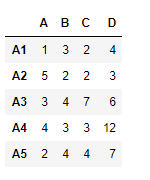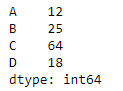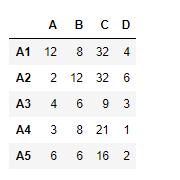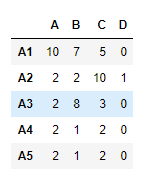# Python | Pandas dataframe.rfloordiv()

Python is a great language for doing data analysis, primarily because of the fantastic ecosystem of data-centric python packages. Pandas is one of those packages and makes importing and analyzing data much easier.

Pandas` dataframe.rfloordiv()` function is used for Integer division of dataframe and other, element-wise (binary operator rfloordiv). This function is essentially same as doing `other // dataframe ` but with a support to substitute for missing data in one of the inputs.

Syntax: DataFrame.rfloordiv(other, axis=’columns’, level=None, fill_value=None)

Parameters :
other : Series, DataFrame, or constant
axis : For Series input, axis to match Series index on
level : Broadcast across a level, matching Index values on the passed MultiIndex leve
fill_value : Fill existing missing (NaN) values, and any new element needed for successful DataFrame alignment, with this value before computation. If data in both corresponding DataFrame locations is missing the result will be missing.

Returns : result : DataFrame

Example #1: Use `rfloordiv()` function to find the integer division of a series with a dataframe.

 `# importing pandas as pd ` `import` `pandas as pd ` ` `  `# Creating the dataframe  ` `df ``=` `pd.DataFrame({``"A"``:[``1``, ``5``, ``3``, ``4``, ``2``], ` `                   ``"B"``:[``3``, ``2``, ``4``, ``3``, ``4``], ` `                   ``"C"``:[``2``, ``2``, ``7``, ``3``, ``4``],  ` `                   ``"D"``:[``4``, ``3``, ``6``, ``12``, ``7``]}, ` `                   ``index ``=``[``"A1"``, ``"A2"``, ``"A3"``, ``"A4"``, ``"A5"``]) ` ` `  `# Print the dataframe ` `df `Let’s create the series

 `# importing pandas as pd ` `import` `pandas as pd ` ` `  `# Create the series ` `sr ``=` `pd.Series([``12``, ``25``, ``64``, ``18``],  ` `     ``index ``=``[``"A"``, ``"B"``, ``"C"``, ``"D"``]) ` ` `  `# Print the series ` `sr `Let’s use the `dataframe.rfloordiv()` function to find the integer division of the series with the dataframe.

 `df.rfloordiv(sr, axis ``=` `1``) `

Output :In the output, a dataframe has been returned, all the elements along the column axis in the dataframe divided the corresponding element in the series.

Example #2: Use `rfloordiv()` function to perform floor division of one dataframe with other.

Note : Here the input dataframe is divided by the dataframe that called the function `dataframe.rfloordiv()`

 `# importing pandas as pd ` `import` `pandas as pd ` ` `  `# Creating the first dataframe  ` `df1 ``=` `pd.DataFrame({``"A"``:[``1``, ``5``, ``3``, ``4``, ``2``], ` `                    ``"B"``:[``3``, ``2``, ``4``, ``3``, ``4``],  ` `                    ``"C"``:[``2``, ``2``, ``7``, ``3``, ``4``],  ` `                    ``"D"``:[``4``, ``3``, ``6``, ``12``, ``7``]},  ` `                    ``index ``=``[``"A1"``, ``"A2"``, ``"A3"``, ``"A4"``, ``"A5"``]) ` ` `  `# Creating the second dataframe ` `df2 ``=` `pd.DataFrame({``"A"``:[``10``, ``11``, ``7``, ``8``, ``5``], ` `                    ``"B"``:[``21``, ``5``, ``32``, ``4``, ``6``],  ` `                    ``"C"``:[``11``, ``21``, ``23``, ``7``, ``9``], ` `                    ``"D"``:[``1``, ``5``, ``3``, ``8``, ``6``]},  ` `                    ``index ``=``[``"A1"``, ``"A2"``, ``"A3"``, ``"A4"``, ``"A5"``]) ` ` `  `# divide df2 by df1 ` `df1.rfloordiv(df2) `

Output :My Personal Notes arrow_drop_upCheck out this Author's contributed articles.

If you like GeeksforGeeks and would like to contribute, you can also write an article using contribute.geeksforgeeks.org or mail your article to contribute@geeksforgeeks.org. See your article appearing on the GeeksforGeeks main page and help other Geeks.

Please Improve this article if you find anything incorrect by clicking on the "Improve Article" button below.

Article Tags :

Be the First to upvote.

Please write to us at contribute@geeksforgeeks.org to report any issue with the above content.